## Using finance

by: Maple

Ibragimova Evelina, 6 class,
school № 57, Kazan

The manual with examples
( templates for the solution of )

The solution of problems on simple interest

> restart:
> with(finance);

[amortization, annuity, blackscholes, cashflows, effectiverate,

futurevalue, growingannuity, growingperpetuity, levelcoupon,

perpetuity, presentvalue, yieldtomaturity]

Team futurevalue (the first installment, rate, period) - the total calculation for a given down payment, interest rate, payments and number of periods.

Example 1. To the Bank account, the income of which is 15% per annum, has made 24 thousand rubles. How many thousands of rubles will be in this account after a year if no transactions on the account will not be carried out? (The answer: 27.60 thousand rubles.)

> futurevalue(260,0.40,1);

364.00

> evalf(1000/216);

> 364*3;

1092

> u:=fsolve(presentvalue(1e6,x,1250)=950,x)*950;

u := 5.303626495

>

Team presentvalue (future amount, rate, period) - the calculation of the initial input to obtain a specified final amount at an interest rate of charges and the number of periods.

Example 2. How much you need to put money in the Bank today, so that when the rate of 27% per annum have in the account after 10 years 100000 thousand rubles? (The answer: 9161.419934 rubles.)

> presentvalue(680,-0.20,1);

850.0000000

The solution of problems in compound interest

The solution of problems
Using commands <futurevalue> и <presentvalue >
> restart;
> with(finance):
Direct task
> futurevalue(,0.,);
`,` unexpected
The inverse problem
> presentvalue(,0.,);
`,` unexpected

I. Case with the same interest rate every period

Using the universal formula F = P*(1+r)^n; , where:
F - the future value (final amount).
P - the initial payment (current amount).
r - the interest rate period.
n - the number of periods.
This formula for the case with the same interest rate every period

> restart:
The task of the formula
> y:=F=P*((1+r)^n):
> y;

n
F = P (1 + r)

The job parameters are known quantities
The interest rate

> r:=;
`;` unexpected
The number of years (periods)
> n:=3;

n := 3

The initial payment (present value)
> P:=;
`;` unexpected
The final amount
> F:=2.16;

F := 2.16

The solution of the equation - the calculation of unknown values (in decimal form)
> `Unknown`;fsolve(y);

Unknown

0

>

II. The case of different interest rates for each period

Formula An = A*(1+1/100*p1)*(1+1/100*p2)*(1+1/100*p3); ... %?(1+1/100*pn); , where
An - the final amount
A - the initial payment (current amount at the moment)
p1, p2, p3, .... pn - interest rate periods
n - the number of periods

> restart:
The task of the formula (need to be adjusted based on the number of periods)
> y:=An=A*(1+1/100*p1)*(1+1/100*p2)*(1+1/100*p3):
> y;

An = A (1 + 1/100 p1) (1 + 1/100 p2) (1 + 1/100 p3)

The task of the parameters of the known values
The initial payment (present value)
> A:=;
`;` unexpected
Interest rate periods
p1:=0.30;
p2:=0.10;
p3:=0.15;

p1 := .30

p2 := .10

p3 := .15

The final amount
> An:=;
`;` unexpected
The solution of the equation - the calculation of unknown values (in decimal form)
> `Unknown`;fsolve(y);

Unknown

0

>

## Opening Matryoshka

by: Maple

Ibragimova Evelina, 6th form,
school № 57, KazanMatreshka.mws

## Units Converter

by: Maple

Ibragimova Evelina, the 6th form

school # 57, Kazan, Russia

The Units Converter

restart:
`Conversion formula`;
d:=l=n*m:
d;

Conversion formula
l = n m

m - shows how many minor units in one major one (coefficient)
`Coefficient`;
m:=1000;
Coefficient
m:=1000

n - the number of major units
n:=7/3;
n := 7/3

l - the number of minor units
l:=;

The result (the desired value)
solve(d);
7000/3
evalf(solve(d));
2333.333333

That is : there are 2333.3 (or 7000/3 ) minor units in 7/3 major units .

Other example

m - shows how many minor units in one major one (coefficient)
`Coefficient`;
m:=4200;
Coefficient
m:=4200

n - the number of major units
n:=;

l - the number of minor units
l:=100;

l:=100

The result (the desired value)
solve(d);
1/42
evalf(solve(d));
0.02380952381

That is : there are 0.02 (or 1/42) major units in 100 minor units .

Another example

m - shows how many minor units in one major one (coefficient)
`Coefficient`;
m:=;
Coefficient

n - the number of major units
n:=2;

n := 2

l - the number of minor units
l:=200;

l:=200

The result (the desired value)
solve(d);
100
evalf(solve(d));
100

That is : Coefficient is 100 .

## Construction of standard quadrilaterals

by: Maple

Construction of standard quadrilaterals

Muchametshina Liya,  8th class,  school № 57, Kazan, Russia

Square

Rectangle

Rhombus

Parallelogram

Trapeze

Construction of square

> restart:
> with(plottools):
Сoordinates (x;y) of the lower left corner of the square and the side "а"
> x:=0;y:=3;a:=6;

x := 0

y := 3

a := 6

Construction of the square
> P1:=plot([[x,y],[x,y+a],[x+a,y+a],[x+a,y],[x,y]],color=green,thickness=4):
> plots[display](P1,scaling=CONSTRAINED);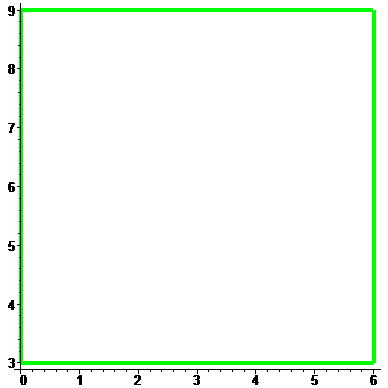The setting of the second square wich moved relative to the first on the vector (2;-3) (vector can be changed) and with side "а-1" (the length of a side can be changed)P2:=plot([[x+2,y-3],[x+2,y-3+a-1],[x+2+a-1,y-3+a-1],[x+2+a-1,y-3],[x+2,y-3]],color=black,thickness=4):
> plots[display](P1,P2,scaling=CONSTRAINED);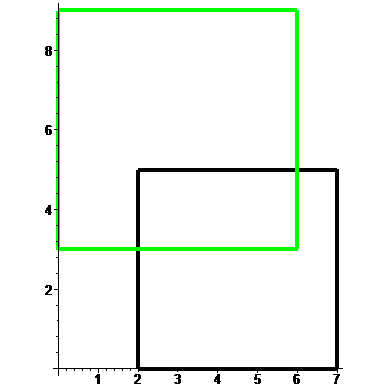Construction of rectangle

> restart:
> with(plottools):
Сoordinates (x;y) of the lower left corner of the square and the "а" and "b" sides
> x:=0;y:=2;a:=3;b:=9;
>

x := 0

y := 2

a := 3

b := 9

The rectangle is specified by the sequence of vertices with given the lengths "a" and "b"
> l:=plot([[x,y],[x,y+a],[x+b,y+a],[x+b,y],[x,y]]):
> plots[display](l,scaling=CONSTRAINED,thickness=4);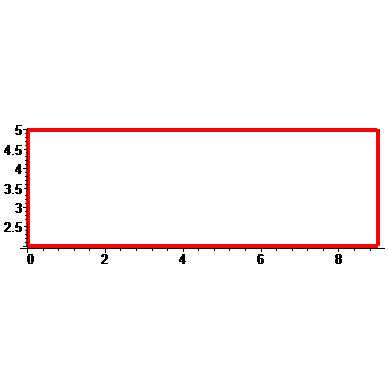Construction of rhombus

> restart:
> with(plottools):
The coordinates (x;y) of the initial vertex of the rhombus and the half of the diagonals "a" and "b"
> x:=0;y:=2;a:=3;b:=4;

x := 0

y := 2

a := 3

b := 4

Rhombus is specified by the sequence of vertices with the values "a" and "b"
> ll:=plot([[x,y],[x+a,y+b],[x+a+a,y],[x+a,y-b],[x,y]]):
> plots[display](ll,scaling=CONSTRAINED,thickness=4);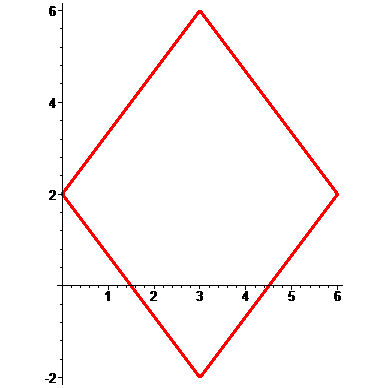Construction of parallelogram

> restart:
> with(plottools):
(х;у) - the starting point, (i;j) - the displacement vector of starting point, "а" - the base of the parallelogram
> x:=0;y:=0;i:=4;j:=5;a:=10;

x := 0

y := 0

i := 4

j := 5

a := 10

The parallelogram is defined by the sequence of vertices
> P1:=plot([[x,y],[x+i,y+j],[x+i+a,y+j],[x+a,y],[x,y]]):
> plots[display](P1,scaling=CONSTRAINED,thickness=4);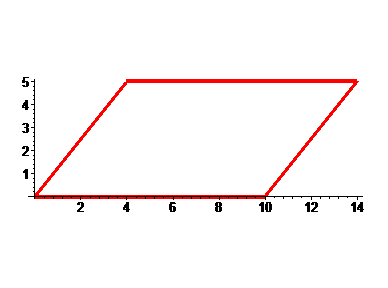If  i= 0  it turns out the rectangleget.
If  j= а  it turns out the  square.
If  a := sqrt(i^2+j^2) it turns out the rhombus. a:=sqrt(i^2+j^2):

Construction of trapeze

Trapeze general form
> restart:
> with(plottools):
>
(х;у) - the starting point, (i;j) - the displacement vector of starting point, а - the larger base of the trapezoid
> x:=0;y:=2;i:=1;j:=5;a:=11;

x := 0

y := 2

i := 1

j := 5

a := 11

The trapez is defined by the sequence of vertices
> P1:=plot([[x,y],[x+i,y+j],[x+i+j,y+j],[x+i+a,y],[x,y]]):
> plots[display](P1,scaling=CONSTRAINED,thickness=4);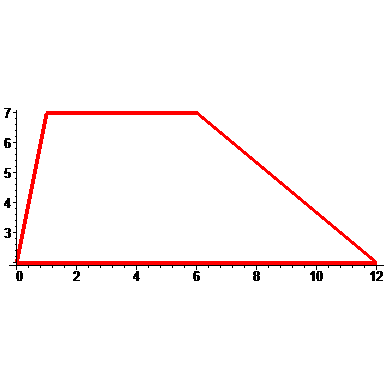Rectangular trapezoid
> restsrt:
> with(plottools):
> x:=0;y:=2;i:=0;j:=6;a:=11;

x := 0

y := 2

i := 0

j := 6

a := 11

> P1:=plot([[x,y],[x,y+j],[x+j,y+j],[x+a,y]]):
> plots[display](P1,scaling=CONSTRAINED,thickness=4);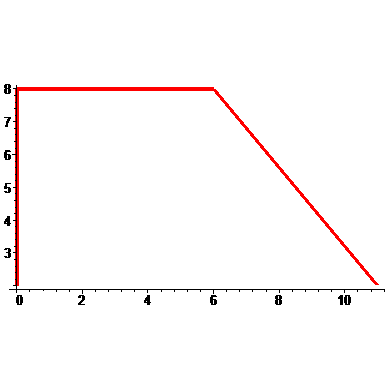Isosceles trapezoid
> restart:
> with(plottools):
> x:=0;y:=2;i:=4;j:=6;a:=15;

x := 0

y := 2

i := 4

j := 6

a := 15

> P1:=plot([[x,y],[x+i,y+j],[x+j+i,y+j],[x+a,y],[x,y]]):
> plots[display](P1,scaling=CONSTRAINED,thickness=4);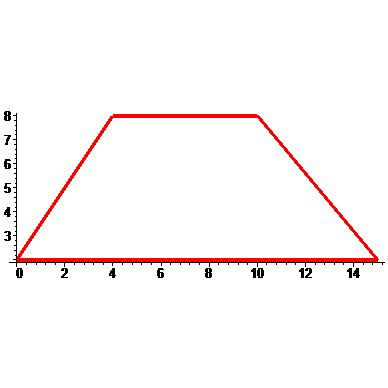## Gialid_Plots_transformation

by: Maple MaplePrimes

> restart;
> a := -10; b := 10; ps := seq(plot([i, t, t = -20 .. 20], x = -10 .. 10, y = -20 .. 20, color = red, style = point), i = a .. b);

plots[display](ps, insequence = true); p := plots[display](ps, insequence = true);restart:
with(plots):
y=sin(x);
p:=implicitplot(y=sin(x),x=-10..10,y=-2..2,thickness=4,color=red,scaling=constrained,numpoints=1000):
plots[display](p);y=sin(3*x);
p0:=implicitplot(y=sin(x),x=-10..10,y=-5..5,thickness=3,color=red,scaling=constrained,numpoints=1000,linestyle=2,style=POINT,symbol=CROSS):
p1:=implicitplot(y=sin(3*x),x=-10..10,y=-5..5,thickness=4,color=blue,numpoints=10000):
plots[display](p0,p1);
y=sin(1/3*x);
p11:=implicitplot(y=sin(1/3*x),x=-10..10,y=-5..5,thickness=4,color=navy,numpoints=10000):
plots[display](p0,p11);y=2*sin(x);
p2:=implicitplot(y=2*sin(x),x=-10..10,y=-5..5,thickness=4,color=blue,numpoints=10000):
plots[display](p0,p2);
y=1/2*sin(x);
p22:=implicitplot(y=1/2*sin(x),x=-10..10,y=-5..5,thickness=4,color=navy,numpoints=10000):
plots[display](p0,p22);y=2+sin(x);
p3:=implicitplot(y=2+sin(x),x=-10..10,y=-5..5,thickness=4,color=blue,numpoints=10000):
plots[display](p0,p3);
y=sin(x)-2;
p33:=implicitplot(y=sin(x)-2,x=-10..10,y=-5..5,thickness=4,color=navy,numpoints=10000):
plots[display](p0,p33);y=sin(x+2);
p4:=implicitplot(y=sin(x+2),x=-10..10,y=-5..5,thickness=4,color=blue,numpoints=10000):
plots[display](p0,p4);
y=sin(x-2);
p44:=implicitplot(y=sin(x-2),x=-10..10,y=-5..5,thickness=4,color=navy,numpoints=10000):
plots[display](p0,p44);y=-sin(x);
p7:=implicitplot(y=-sin(x),x=-10..10,y=-5..5,thickness=4,color=blue,numpoints=10000):
plots[display](p0,p7);
y=sin(-x);
p77:=implicitplot(y=sin(-x),x=-10..10,y=-5..5,thickness=4,color=navy,numpoints=10000):
plots[display](p0,p77);y=abs(sin(x));
p00:=implicitplot(y=sin(x),x=-10..10,y=-5..5,thickness=3,color=red,scaling=constrained,numpoints=1000,linestyle=2,style=POINT,symbol=BOX):
p5:=implicitplot(y=abs(sin(x)),x=-10..10,y=-5..5,thickness=4,color=blue,numpoints=10000):
plots[display](p00,p5);
plots[display](p5,scaling=constrained);y=sin(abs(x));
p00:=implicitplot(y=sin(x),x=-10..10,y=-5..5,thickness=3,color=red,scaling=constrained,numpoints=1000,linestyle=2,style=POINT,symbol=BOX):
p6:=implicitplot(y=sin(abs(x)),x=-10..10,y=-5..5,thickness=4,color=navy,numpoints=10000):
plots[display](p00,p6);
plots[display](p6,scaling=constrained);## Russian secondary school Maple project

by: Maple

Post gialid_GEODROMchik - what is this?

Pilot project of Secondary school # 57 of Kazan, Russia

Use of Maple

in Mathematics Education by mathematics teacher Alsu Gibadullina

and in scientific work of schoolchildren

Examples made using the Maple

the 6th class

Arina                         Elza                             DavidArtur## From combinatorics: an algorithm to enumerate...

by: Maple

Greetings to all. I am writing today to share a personal story / exploration using Maple of an algorithm from the history of combinatorics. The problem here is to count the number of strings over a certain alphabet which consist of some number of letters and avoid a set of patterns (these patterns are strings as opposed to regular expressions.) This counting operation is carried out using rational generating functions that encode the number of admissible strings of length n in the coefficients of their series expansions. The modern approach to this problem uses the Goulden-Jackson method which is discussed, including a landmark Maple implementation from a paper by D. Zeilberger and J. Noonan, at the following link at math.stackexchange.com (Goulden-Jackson has its own website, all the remaining software described in the following discussion is available at the MSE link.) The motivation for this work was a question at the MSE link about the number of strings over a two-letter alphabet that avoid the pattern ABBA.

As far as I know before Goulden-Jackson was invented there was the DFA-Method (Deterministic Finite Automaton also known as FSM, Finite State Machine.) My goal in this contribution was to study and implement this algorithm in order to gain insight about its features and how it influenced its powerful successor. It goes as follows for the case of a single pattern string: compute a DFA whose states represent the longest prefix of the pattern seen at the current position in the string as it is being scanned by the DFA, with the state for the complete pattern doubling as a final absorbing state, since the pattern has been seen. Translate the transitions of the DFA into a system of equations in the generating functions representing strings ending with a given maximal prefix of the pattern, very much like Markov chains. Finally solve the system of equations for the generating functions and thus obtain the sequence of values of strings of length n over the given alphabet that avoid the given pattern.

I have also implemented the DFA method for sets of patterns as opposed to just one pattern. The algorithm is the same except that the DFA does not consist of a chain with backlinks as in the case of a single pattern but a tree of prefixes with backlinks to nodes higher up in the tree. The nodes in the tree represent all prefixes that need to be tracked where obviously a common prefix between two or more patterns is shared i.e. only represented once. The DFA transitions emanating from nodes that are leaves represent absorbing states indicating that one of the patterns has been seen. We run this algorithm once it has been verified that the set of patterns does not contain pairs of patterns where one pattern is contained in another, which causes the longer pattern to be eliminated at the start. (Obviously if the shorter pattern is forbidden the so is the longer.) The number of states of the DFA here is bounded above by the sum of the lengths of the patterns with subpatterns eliminated. The uniqueness property of shared common prefixes holds for subtrees of the main tree i.e. recursively. (The DFA method also copes easily with patterns that have to occur in a certain order.)

I believe the Maple code that I provide here showcases many useful tricks and techniques and can help the reader advance in their Maple studies, which is why I am alerting you to the web link at MSE. I have deliberately aimed to keep it compatible with older versions of Maple as many of these are still in use in various places. The algorithm really showcases the power of Maple in combinatorics computing and exploits many different aspects of the software from the solution of systems of equations in rational generating functions to the implementation of data structures from computer science like trees. Did you know that Maple permits nested procedures as known to those who have met Lisp and Scheme during their studies? The program also illustrates the use of unit testing to detect newly introduced flaws in the code as it evolves in the software life cycle.

Enjoy and may your Maple skills profit from the experience!

Best regards,

Marko Riedel

The software is also available here: dfam-mult.txt

## Visualizing Multiple Quandl Data Sets using Bubble...

by: Maple 2015

This is the first of three blog posts about working with data sets in Maple.

In 2013, I wrote a library for Maple that used the HTTP package to access the Quandl data API and import data sets into Maple. I was motivated by the fact that, when I was downloading data, I often used multiple data sources, manually updated data when updates were available, and cleaned or manipulated the data into a standardized form (which left me spending too much time on the data acquisition step).

Simply put, I needed a source for data that would provide me with a searchable, stable data API, which would also return data in a form that did not require too much post-processing.

My initial library had really just scratched the surface of what was possible.

Maple 2015 introduced the new DataSets package, which fully integrated a data set search into core library routines and made its functionality more discoverable through availability in Maple’s search bar.

Accessing online data suddenly became much easier. From within Maple, I could now search through over 12 million time series data sets provided by Quandl, and then automatically import the data into a format that I could readily work with.

If you’re not already aware of this online service, Quandl is an online data aggregator that delivers a wide variety of high quality financial and economic data. This includes the latest data on stocks and commodities, exchange rates, and macroeconomic indicators such as population, inflation, unemployment, and so on. Quandl collects both open and proprietary data sets from many sources, such as the US Federal Reserve System, OECD, Eurostat, The World Bank, and Open Data for Africa. Best of all, Quandl's powerful API is free to use.One of the first examples for the DataSets package that I constructed was in part based on the inspirational work of Hans Rosling. I was drawn in by his ability to use statistical visualizations to break down complex multidimensional data sets and provide insight into underlying patterns; a key example investigating the correlation between rising incomes and life expectancy.

As well as online data, the DataSets package had a database for country data. Hence it seemed fitting to add an example that explored macroeconomic indicators for several countries. Accordingly, I set out to create an example that visualized variables such as Gross Domestic Product, Life Expectancy, and Population for a collection of countries.

I’ll now describe how I constructed this application.

The three key variables are Gross Domestic Product at Power Purchasing Parity, Life Expectancy, and Population. Having browsed through Quandl’s website for available data sets, the World Bank and Open Data for Africa projects seemingly had the most available relevant data; therefore I chose these as my data sources.

Pulling data for a single country from one of these sources was pretty straight forward. For example, the DataSets Reference for the Open Data for Africa data set on GDP at PPP for Canada is:

`DataSets:-Reference("quandl", "ODA/CAN_PPPPC"));`

In this command, the second argument is the Quandl data set code. If you are on Quandl’s website, this is listed near the top of the data set page as well as in the last few characters of the web address itself: https://www.quandl.com/data/ODA/CAN_PPPPC . Deconstructing the code, “ODA” stands for Open Data for Africa and the rest of the string is constructed from the three letter country code for Canada, “CAN”, and the code for the GDP and PPP. Looking at a small sample of other data set codes, I theorized that both of the data sources used a standardized data set name that included the ISO-3166 3-letter country code for available data sets. Based on this theory, I created a simple script to query for available data and discovered that there was data available for many countries using this standardized code. However, not every country had available data, so I needed to filter my list somewhat in order to pick only those countries for which information was available.

The script that I had constructed required three letter country codes. In order to test all available countries, I created a table to house the country names and three-letter country codes using data from the built-in database for countries:

`ccdata := DataSets:-Builtin:-Reference("country")[.., "3 Letter Country Code"];`
`cctable := table([seq(op(GetElementNames(ccdata[i])) = ccdata[i, "3 Letter Country Code"],                                 i = 1 .. CountRows(ccdata))]):`

My script filtered this table, returning a subset of the original table, something like:

`Countries := table( [“Canada” = “CAN”, “Sweden” = “SWE”, … ] );`

You can see the filtered country list in the code edit region of the application below.

With this shorter list of countries, I was now ready to download some data. I created three vectors to hold the data sets by mapping in the DataSets Reference onto the “standardized” data set names that I pulled from Quandl. Here’s the first vector for the data on GDP at PPP.

`V1 := Vector( [ (x) -> Reference("quandl", cat("ODA/", x, "_PPPPC"))`
`                   ~([entries(Countries, nolist, indexorder)])]):`
`#Open Data for Africa GDP at PPP`

Having created three data vectors consisting of 180 x 3 = 540 data sets, I was finally ready to visualize the large set of data that I had amassed.

In Maple’s Statistics package, BubblePlots can use the horizontal axis, vertical axis and the relative bubble size to illustrate multidimensional information. Moreover, if incoming data is stored as a TimeSeries object, BubblePlots can generate animations over a common period of time.

Putting all of this together generated the following animation for 180 available countries.This example will be included with the next version of Maple, but for now, you can download a copy here:DataSetsBubblePlot.mw

*Note: if you try this application at home, it will download 540 data sets. This operation plus the additional BubblePlot construction can take some time, so if you just want to see the finished product, you can simply interact with the animation in the Maple worksheet using the animation toolbar.

A more advanced example that uses multiple threads for data download can be seen at the bottom of the following page: https://www.maplesoft.com/products/maple/new_features/maple19/datasets_maple2015.pdf You can also interact with this example in Maple by searching for: ?updates,Maple2015,DataSets

In my next post, I’ll discuss how I used programmatic content generation to construct an interactive application for data retrieval.

## Announcing Maple 2016

by: Maple 2016You, I, and others like us, are the beneficiaries of decades of software evolution.

From its genesis as a research project at the University of Waterloo in the early 80s, Maple has continually evolved to meet the challenges of technical computing.

## Bring Learning to Life (LIVE!)

by: Maple

This January 28th, we will be hosting another full-production, live streaming webinar featuring an all-star cast of Maplesoft employees: Andrew Rourke (Director of Teaching Solutions), Jonny Zivku (Maple T.A. Product Manager), and Daniel Skoog (Maple Product Manager). Attend the webinar to learn how educators all around the world are using Maple and Maple T.A. in their own classrooms.

Any STEM educator, administrator, or curriculum coordinator who is interested in learning how Maple and Maple T.A. can help improve student grades, reduce drop-out rates, and save money on administration costs will benefit from attending this webinar.

Click here for more information and registration.

## Finite Element Analysis

by: Maple 2015

I am learning to use maple for my notes preparation for the subject Finite Element Analysis. It is interesting to know that how often we blame maple or computer for the silly mistakes we made in our commands and expect the exact answers. I have used a small file and find it easy to analyse my mistakes fatser. If we make a small mistake in a big file, it not only gives us problem finding our mistakes, it leads to more mistakes in other parts as well. A command working in one document need not necessarily work the same way in other document.

I have made my first document and people will come with suggestions to make appropriate modifications in the various sections to improve my knowledge on maple as well as the subject.

Download FINITE_ELEMENT_ANALYSIS.mw

Ramakrishnan V

rukmini_ramki@hotmail.com

## An Arts Co-op Student in a Math Software Company

by: MapleSim

As an Arts major at the University of Waterloo, my first day as a co-op student in the Maplesoft marketing department was a bit of a blur. I was hearing a lot of mathematical jargon that I did not understand. Other than a mandatory statistics class in my second year at university, I haven’t taken a math course since high school, over two years ago. I spent my first week as the marketing assistant educating myself about the basics of marketing complex math software. My favourite method for doing this was to read through the Maplesoft user stories. As I read, I was amazed by the variety of customers and the endless applications that Maplesoft products had contributed to. It became apparent that math is a part of every industry and it is in the design of many products. There were a few stories from the robotics industry in particular that really sparked my interest in the software that I now market.

We’ve all seen the futuristic movies where robots gradually get smarter and smarter, developing enough intelligence to control the human race, and eventually, take over the world. As it turns out, Engineered Arts, a UK robotics company, is bringing us one step closer to that reality. Well… they’re maybe not ready for world domination just yet, but they are working on one of the most advanced and human-like robots that the world has seen outside of a Hollywood production, and they are doing this using MapleSim. The first generation of the biologically inspired robot was named RoboThespian. With his ability to speak and sing, he was used to educate, entertain, and investigate new developments in robotics. However, he was largely static. That’s when the engineers began work on generation two of their robot, named Byrun, who has the ability to walk, run, jump, and hop as well as speak and sing. Byrun can even express thousands of different facial features thanks to his projective head display. This makes him even more human-like; scary or cool? I’m thinking a bit of both. If you’re interested in the story, click here to continue reading about it.

Another unexpected use of MapleSim was adopted as a joint research project between Ryerson University and McMaster University. I never would have guessed that math software could be applied to the process of human birth. Nevertheless, a group of researchers used MapleSim to simulate induced labour with a Foley Catheter. In short, this is when a small balloon is inserted through the opening of the cervix creating a downward pressure that effectively tricks the cervix into opening for labour to begin. Though the application of this story surprised me, it makes a lot of sense to use modelling software for a research project like this. It’s more efficient to get all of the kinks out of the virtual model in a simulation program before building a physical model that could end up being dysfunctional. According to Dr. James Andrew Smith, a Biomedical Engineering researcher and Assistant Professor in Electrical and Computer Engineering, who is the lead researcher on the project, “Modern engineering has a lot to offer the medical world,” especially when it saves on time and cost. Click here to read more about this story and to watch a video of the finished model.

After two months at Maplesoft, I have noticed that I don’t look at things in the same way that I used to. I find myself staring at a toaster and imagining how it was designed. Did the engineers use advanced physical simulation and modeling software to make the most efficient toaster possible? Well, if it can still only toast on one side then, my guess is no! Maplesoft has many more user stories that I haven’t had the chance to read yet. With customers ranging from BMW to Pixar, Maplesoft continues to expand its customer base and adapt its software to support more and more unique applications. I can’t wait to hear what new and unexpected things will be done with the software next!

## In-depth Design Using Multidimensional Visualizati...

by: Maple

The engineering design process involves numerous steps that allow the engineer to reach his/her final design objectives to the best of his/her ability. This process is akin to creating a fine sculpture or a great painting where different approaches are explored and tested, then either adopted or abandoned in favor of better or more developed and fine-tuned ones. Consider the x-ray of an oil painting. X-rays of the works of master artists reveal the thought and creative processes of their minds as they complete the work. I am sure that some colleagues may disagree with the comparison of our modern engineering designs to art masterpieces, but let me ask you to explore the innovations and their brilliant forms, and maybe you will agree with me even a little bit.Design Process

Successful design engineers must have the very best craft, knowledge and experience to generate work that is truly worthy of being incorporated in products that sell in the tens, or even hundreds, of millions. This is presently achieved by having cross-functional teams of engineers work on a design, allowing cross checking and several rounds of reviews, followed by multiple prototypes and exhaustive preproduction testing until the team reaches a collective conclusion that “we have a design.” This is then followed by the final design review and release of the product. This necessary and vital approach is clearly a time consuming and costly process. Over the years I have asked myself several times, “Did I explore every single detail of the design fully”? “Am I sure that this is the very best I can do?” And more importantly, “Does every component have the most fine-tuned value to render the best performance possible?” And invariably I am left with a bit of doubt. That brings me to a tool that has helped me in this regard.

A Great New Tool

I have used Maple for over 25 years to dig deeply into my designs and understand the interplay between a given set of parameters and the performance of the particular circuit I am working on. This has always given me a complete view of the problem at hand and solidly pointed me in the direction of the best possible solutions.

In recent years, a new feature called “Explore” has been added to Maple. This amazing feature allows the engineer/researcher to peer very deeply into any formula and explore the interaction of EVERY variable in the formula.

Take for example the losses in the control MOSFET in a synchronous buck converter. In order to minimize these losses and maximize the power conversion efficiency, the most suitable MOSFET must be selected. With thousands of these devices being available in the market, a dozen of them are considered very close to the best at any given time. The real question then is, which one is really the very best amongst all of them?

There are two possible approaches - one, build an application prototype, test a random sample of each and choose the one that gives you the best efficiency.  Or, use an accurate mathematical model to calculate the losses of each and chose the best. The first approach lacks the variability of each parameter due to the six sigma statistical distribution where it is next to impossible to get a device laying on the outer limits of the distribution. That leaves the mathematical model approach. If you take this route, you can have built-in tolerances in the equations to accommodate all the variabilities and use a simplified equation for the control MOSFET losses (clearly you can use a very detailed model should you chose to) to explore these losses. Luckily you can explore the losses using the Explore function in Maple.

The figure below shows a three dimensional plot, plus five other variables in the formula that the user can change using sliders that cover the range of values of interest including Minima and Maxima, while observing in real time the effects of the change on the power loss.This means that by changing the values of any set of variables, you can observe their effect on the function. To put it simply, this single feature helps you replace dozens of plots with just one, saving you precious time and cost in fine-tuning your design. In my opinion, this is equivalent to an eight-dimensional/axes plot.

I used this amazing feature in the last few weeks and I was delighted at how simple it is to use and how much it simplifies the study of my approach and my components selection, in record times!

## Spam is all around

MaplePrimes

Hello!

The last ten posts I see are spam!

Isn't it possible to stop them from spaming this Forum?

Best,

Jean-Michel

## Comparisons

Maple

Hello all ,

Having read a recent post about comparing Maple and Mathematica I'd like to throw my 2 cents (FWIW).

It is *silly* (not to say stupid) to compare these two softwares.

Maple can do "things" that Mathematica can't. For examples Differential Geometry, Lie Algrebra, covariant derivative and the like.

And Mathematica Manipulate command is far better than Maple Explore (just another exemple).

I have being using Maple since Release V.2 (1992) and Mathematica since Release 1.1.a (1991).

I use both of them on a daily basis and I *LOVE* them both.

Inputs are welcome :-)

Kind regards to all,

Jean-Michel.

﻿